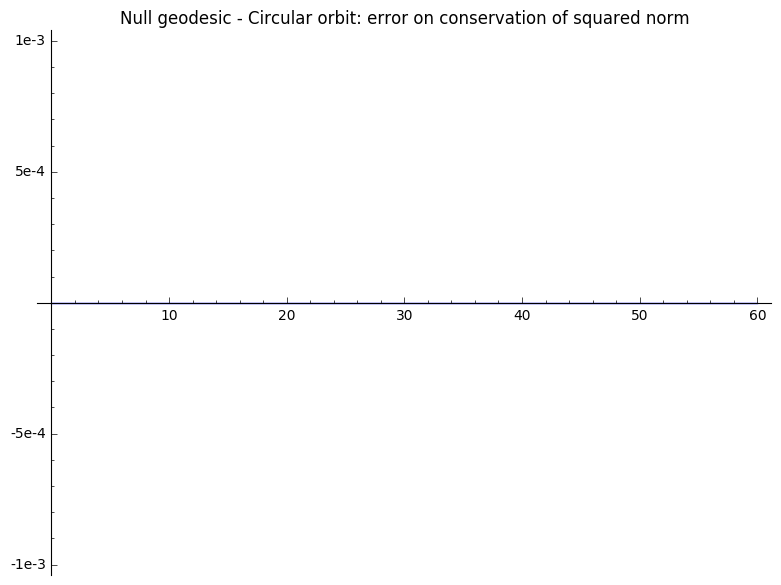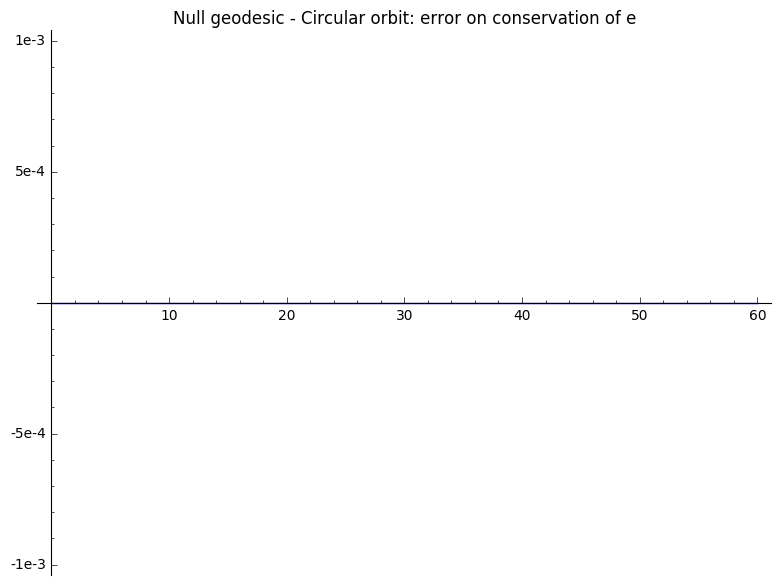# Geodesics in Schwarzschild spacetime¶

This worksheet aims at recovering some geodesics of Schwarzschild spacetime using numerical tools implemented in the SageMath class IntegratedGeodesic, within the SageManifolds project (version 1.1, as included in SageMath 8.1).

Click here to download the worksheet file (ipynb format). To run it, you must start SageMath within the Jupyter notebook, via the command sage -n jupyter

NB: a version of SageMath at least equal to 8.1 is required to run this worksheet:

In :
version()

Out:
'SageMath version 8.1, Release Date: 2017-12-07'

First set up the notebook to display mathematical objects using LaTeX rendering:

In :
%display latex


## Spacetime manifold¶

To set the Schwarzschild spacetime, declare a 4-dimensional differentiable manifold and a non-negative parameter $m$:

In :
Schw = Manifold(4, 'Schw', r'\mathcal{Schw}')
m = var('m') ; assume(m >= 0)


Define the Boyer-Lindquist coordinates on the union of two subregions $\mathcal{R}_{\mathrm{I}}$ and $\mathcal{R}_{\mathrm{II}}$:

In :
regI = Schw.open_subset('R_I', r'\mathcal{R}_{\mathrm{I}}')
regII = Schw.open_subset('R_II', r'\mathcal{R}_{\mathrm{II}}')
regI_II = regI.union(regII)
BL.<t,r,th,ph> = regI_II.chart(r't r:(0,+oo) th:(0,pi):\theta ph:\phi')
BL_I = BL.restrict(regI, r>2*m)
BL_II = BL.restrict(regII, r<2*m)


Finally set the Lorentzian metric of Schwarzschild spacetime:

In :
g = Schw.lorentzian_metric('g')
g[0,0], g[1,1] = -(1-2*m/r), 1/(1-2*m/r)
g[2,2], g[3,3] = r^2, (r*sin(th))^2
g.display()

Out:

## Geodesics in Boyer-Lindquist coordinates¶

### Defining the geodesic¶

Declare the various variables needed to define a geodesic; start with the affine parameter and its extremal values:

In :
affine_param = var('s s_0 s_max')


Then, declare the starting point of the geodesic:

In :
initial_pt_coords = var('t_0 r_0 th_0 ph_0') ; assume(r_0 > 2*m)
p_0 = regI.point(initial_pt_coords, name='p_0')


Declare the initial tangent vector:

In :
initial_tgt_vec_comps = var('Dt_0 Dr_0 Dth_0 Dph_0')
v_0 = Schw.tangent_space(p_0)(initial_tgt_vec_comps)


The parametrised geodesic may now be initialised:

In :
geod = Schw.integrated_geodesic(g, affine_param, v_0, verbose=True)

The curve was correctly set.
Parameters appearing in the differential system defining the curve are [Dph_0, Dr_0, Dt_0, Dth_0, m, ph_0, r_0, s_0, s_max, t_0, th_0].


Display the system of the geodesic equations:

In :
sys = geod.system(verbose=True)

Geodesic in the 4-dimensional differentiable manifold Schw equipped with Lorentzian metric g on the 4-dimensional differentiable manifold Schw, and integrated over the Real interval (s_0, s_max) as a solution to the following geodesic equations, written with respect to Chart (R_I_union_R_II, (t, r, th, ph)):

Initial point: Point p_0 on the 4-dimensional differentiable manifold Schw with coordinates [t_0, r_0, th_0, ph_0] with respect to Chart (R_I_union_R_II, (t, r, th, ph))
Initial tangent vector: Tangent vector at Point p_0 on the 4-dimensional differentiable manifold Schw with components [Dt_0, Dr_0, Dth_0, Dph_0] with respect to Chart (R_I_union_R_II, (t, r, th, ph))

d(t)/ds = Dt
d(r)/ds = Dr
d(th)/ds = Dth
d(ph)/ds = Dph
d(Dt)/ds = 2*Dr*Dt*m/(2*m*r - r^2)
d(Dr)/ds = -(4*Dth^2*m^2*r^3 - 4*Dth^2*m*r^4 + Dth^2*r^5 - 4*Dt^2*m^3 + 4*Dt^2*m^2*r + (Dr^2 - Dt^2)*m*r^2 + (4*Dph^2*m^2*r^3 - 4*Dph^2*m*r^4 + Dph^2*r^5)*sin(th)^2)/(2*m*r^3 - r^4)
d(Dth)/ds = (Dph^2*r*cos(th)*sin(th) - 2*Dr*Dth)/r
d(Dph)/ds = -2*(Dph*Dth*r*cos(th) + Dph*Dr*sin(th))/(r*sin(th))



### Computing numerical solutions¶

#### Timelike geodesics¶

##### Bounded orbit¶

Set a dictionnary providing numerical values for each of the parameters apprearing in the system defining the geodesic.

The values suggested below make the initial tangent vector timelike with squared norm equal to -1. This way, the curve parameter s not only is an affine parameter of the (timelike) geodesic, but it actually is the proper time along it (up to numerical roundings).

In :
params_values_bounded = {m:1, s_0:0, s_max:1500, t_0:0, r_0:8, th_0:pi/2, ph_0:1e-12,
Dt_0:sqrt(80.81)/(4*sqrt(3)), Dr_0:0, Dth_0:0, Dph_0:4.1/64}


Then integrate the geodesic for such values of the parameters:

In :
Schw.default_chart().valid_coordinates(*p_0.coord())

Out:
In :
sol_bounded = geod.solve(step=4, parameters_values=params_values_bounded,
solution_key='timelike_bounded_equatorial', verbose=True)

Performing numerical integration with method 'rk4_maxima'...
Numerical integration completed.

Checking all points are in the chart domain...
All points are in the chart domain.

The resulting list of points was associated with the key 'timelike_bounded_equatorial' (if this key already referred to a former numerical solution, such a solution was erased).


The squared norm $g_{\mu\nu} \dot{x}^{\mu} \dot{x}^{\nu}$ of the vector tangent to any geodesic with respect to any affine parameter $s$ is constant throughout motion.

In addition, up to a rotation of the system of polar coordinates, any geodesic in Schwarzschild spacetime is known to have constant coordinate $\theta$ equal to $\pi/2$ (which is why it was suggested above to set the initial condition on $\theta$ to $\pi/2$). In such a situation, energy $e$ and angular momentum $l$ per unit mass, defined as follows, are also conserved along a geodesic of Schwarzschild spacetime:

$$e = \left( 1 - \frac{2m}{r} \right) \dot{t}$$$$l = r^{2} \dot{\phi}$$

Therefore, using an interpolation of the previous numerical solution, one may check that these three quantities are indeed conserved along the numerical solution previoulsy computed (not to be disturbed by initial edge effects, the reference value used to check conservations of the various quantities is updated after 10 steps):

In :
interp_bounded = geod.interpolate(solution_key='timelike_bounded_equatorial',
interpolation_key='timelike_bounded_equatorial',
verbose=True)

error_squar_norm_bounded = []
error_e_bounded = []
error_l_bounded = []

i = 0
for (S,T,R,TH,PH) in sol_bounded:
P = geod(S, interpolation_key='timelike_bounded_equatorial')
V = geod.tangent_vector_eval_at(S, interpolation_key='timelike_bounded_equatorial')

squar_norm_bounded = numerical_approx((g.at(P)(V,V)).substitute({m:1}))
e_bounded = numerical_approx((-g.at(P)[0,0]*V).substitute({m:1}))
l_bounded = numerical_approx((g.at(P)[3,3]*V).substitute({m:1}))

if i == 0:
squar_norm_bounded_0 = squar_norm_bounded
e_bounded_0 = e_bounded
l_bounded_0 = l_bounded

if i == 10:
squar_norm_bounded_0 = squar_norm_bounded
e_bounded_0 = e_bounded
l_bounded_0 = l_bounded

error_squar_norm_bounded += [(S,squar_norm_bounded - squar_norm_bounded_0)]
error_e_bounded += [(S,e_bounded - e_bounded_0)]
error_l_bounded += [(S,l_bounded - l_bounded_0)]

i += 1

plot_error_squar_norm_bounded = line(error_squar_norm_bounded)
plot_error_e_bounded = line(error_e_bounded)
plot_error_l_bounded = line(error_l_bounded)

plot_error_squar_norm_bounded.show(title="Timelike geodesic - Bounded orbit: error on conservation of squared norm",
ymin=-1e-3, ymax=1e-3)
plot_error_e_bounded.show(title="Timelike geodesic - Bounded orbit: error on conservation of e",
ymin=-1e-3, ymax=1e-3)
plot_error_l_bounded.show(title="Timelike geodesic - Bounded orbit: error on conservation of l",
ymin=-2e-3, ymax=2e-3)

Performing cubic spline interpolation by default...
Interpolation completed and associated with the key 'timelike_bounded_equatorial' (if this key already referred to a former interpolation, such an interpolation was erased).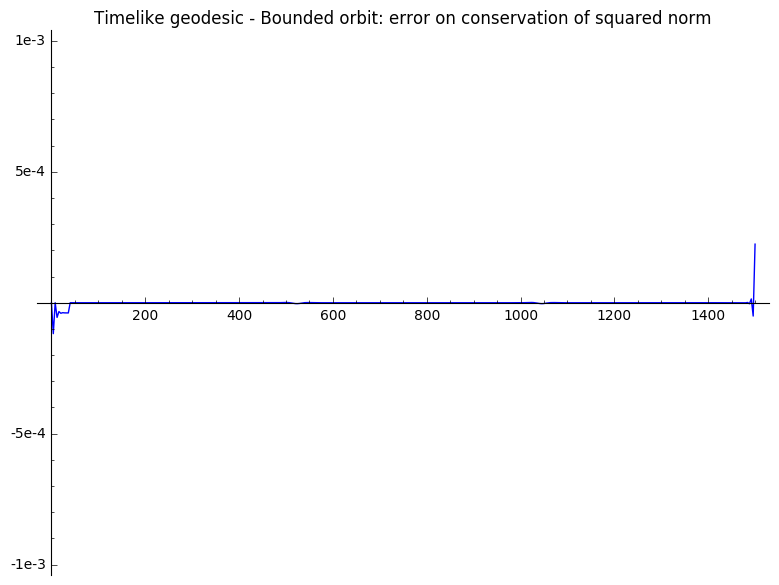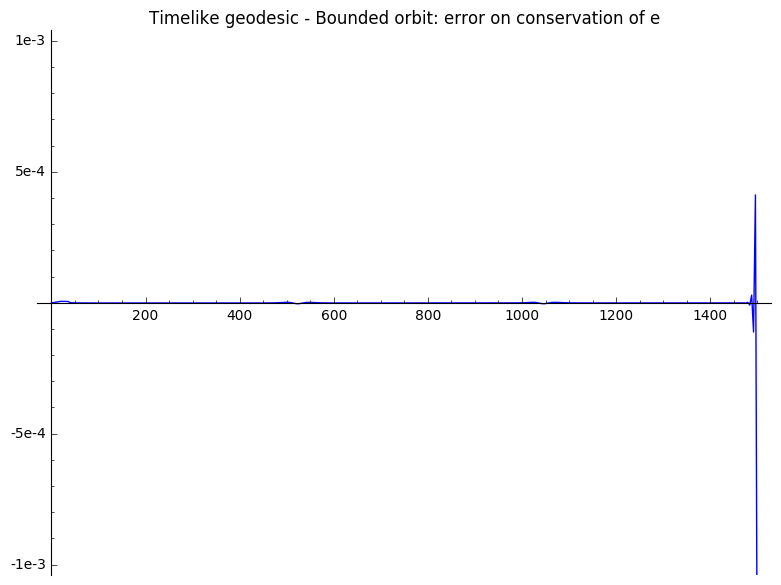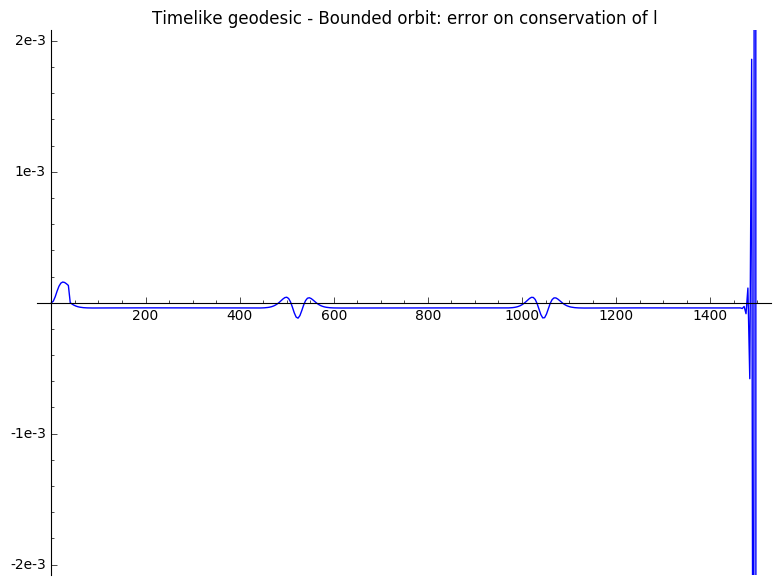The curves are indeed constant with negligible numerical variations, the jolts in the middle being correlated with radial coordinate $r$ reaching a minimum. The jolts get smaller if one uses a shorter step of integration when calling method solve.

One may finally plot the spatial part of the geodesic. To do so, start with defining the 3-dimensional Euclidean space with Cartesian coordinates:

In :
R3 = Manifold(3, 'R3', start_index=1)
cart.<x,y,z> = R3.chart()
origin = R3.point((0,0,0), name='O')
plot3D_origin = origin.plot(size=10)


Then define the mapping converting the spatial part of Boyer-Lindquist coordinates (i.e. the polar coordinates) into the Cartesian coordinates:

In :
BL_spatial_coords = Schw.diff_map(R3,{(BL, cart): [r*sin(th)*cos(ph), r*sin(th)*sin(ph),
r*cos(th)]})


This allows to plot the spatial part of the geodesic:

In :
plot3D_projected_geod_bounded = geod.plot_integrated(interpolation_key='timelike_bounded_equatorial',
mapping=BL_spatial_coords,
plot_points=500, thickness=2,
display_tangent=True,
plot_points_tangent=30, scale=8,
width_tangent=1, label_axes=False)


Plot the event horizon:

In :
plot3D_event_horizon = BL.plot(chart=cart, mapping=BL_spatial_coords,
fixed_coords={t:0, r:2}, ranges={ph:(0,2*pi)},
number_values=15, color='yellow', label_axes=False)


Display all graphics:

In :
(plot3D_projected_geod_bounded + plot3D_event_horizon + plot3D_origin).show(viewer='threejs',
online=True)


As expected, one notices the advance of the periastron of such a bounded orbit.

##### Circular case¶

Schwarzschild spacetime admits circular orbits. The unique timelike geodesic (still parametrised with proper time) inducing a stable circular orbit with same angular momentum as that of the geodesic considered above is obtained with the following initial conditions:

In :
params_values_circular = {m:1, s_0:0, s_max:500, t_0:0, r_0:(16.81 + 4.1*sqrt(4.81))/2,
th_0:pi/2, ph_0:0, Dt_0:1.1415, Dr_0:0, Dth_0:0,
Dph_0:16.4/(16.81+4.1*sqrt(4.81))^2}


Solve, interpolate and plot the orbit corresponding to such values of the parameters:

In :
sol_circular = geod.solve(step=4, parameters_values=params_values_circular,
solution_key='timelike_circular_equatorial', verbose=True)
interp_circular = geod.interpolate(solution_key='timelike_circular_equatorial',
interpolation_key='timelike_circular_equatorial',
verbose=True)

error_squar_norm_circular = []
error_e_circular = []
error_l_circular = []
error_r_circular = []

i = 0
for (S,T,R,TH,PH) in sol_circular:
P = geod(S, interpolation_key='timelike_circular_equatorial')
V = geod.tangent_vector_eval_at(S, interpolation_key='timelike_circular_equatorial')

squar_norm_circular = numerical_approx((g.at(P)(V,V)).substitute({m:1}))
e_circular = numerical_approx((-g.at(P)[0,0]*V).substitute({m:1}))
l_circular = numerical_approx((g.at(P)[3,3]*V).substitute({m:1}))

if i == 0:
squar_norm_circular_0 = squar_norm_circular
e_circular_0 = e_circular
l_circular_0 = l_circular
R_0 = R

error_squar_norm_circular += [(S,squar_norm_circular - squar_norm_circular_0)]
error_e_circular += [(S,e_circular - e_circular_0)]
error_l_circular += [(S,l_circular - l_circular_0)]
error_r_circular += [(S,R - R_0)]

i += 1

plot_error_squar_norm_circular = line(error_squar_norm_circular)
plot_error_e_circular = line(error_e_circular)
plot_error_l_circular = line(error_l_circular)
plot_error_r_circular = line(error_r_circular)

plot_error_squar_norm_circular.show(title=
"Timelike geodesic - Circular orbit: error on conservation of squared norm",
ymin=-1e-3, ymax=1e-3)
plot_error_e_circular.show(title="Timelike geodesic - Circular orbit: error on conservation of e",
ymin=-1e-3, ymax=1e-3)
plot_error_l_circular.show(title="Timelike geodesic - Circular orbit: error on conservation of l",
ymin=-1e-3, ymax=1e-3)
plot_error_r_circular.show(title="Timelike geodesic - Circular orbit: error on conservation of r",
ymin=-1e-3, ymax=1e-3)

plot3D_projected_geod_circular = geod.plot_integrated(interpolation_key='timelike_circular_equatorial',
mapping=BL_spatial_coords,
plot_points=250, thickness=2,
display_tangent=True,
plot_points_tangent=10, scale=8,
width_tangent=1, label_axes=False)

(plot3D_projected_geod_circular + plot3D_event_horizon + plot3D_origin).show(viewer='threejs',
online=True)

Performing numerical integration with method 'rk4_maxima'...
Numerical integration completed.

Checking all points are in the chart domain...
All points are in the chart domain.

The resulting list of points was associated with the key 'timelike_circular_equatorial' (if this key already referred to a former numerical solution, such a solution was erased).
Performing cubic spline interpolation by default...
Interpolation completed and associated with the key 'timelike_circular_equatorial' (if this key already referred to a former interpolation, such an interpolation was erased).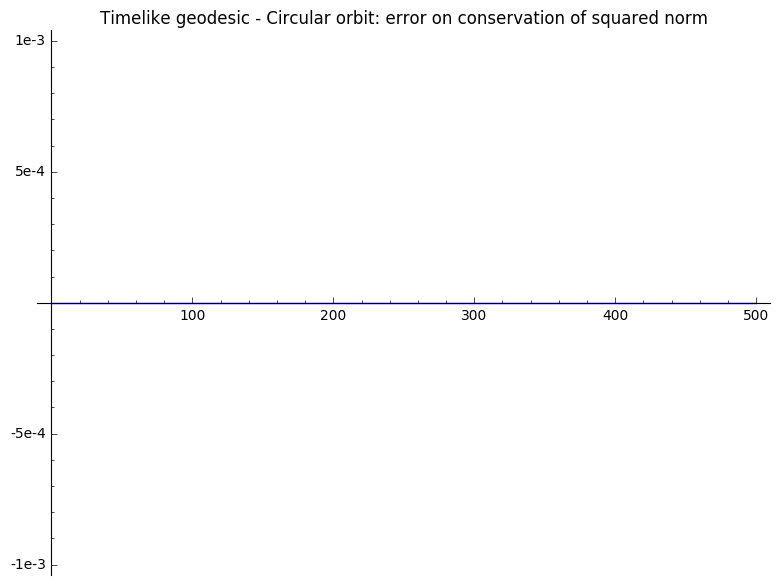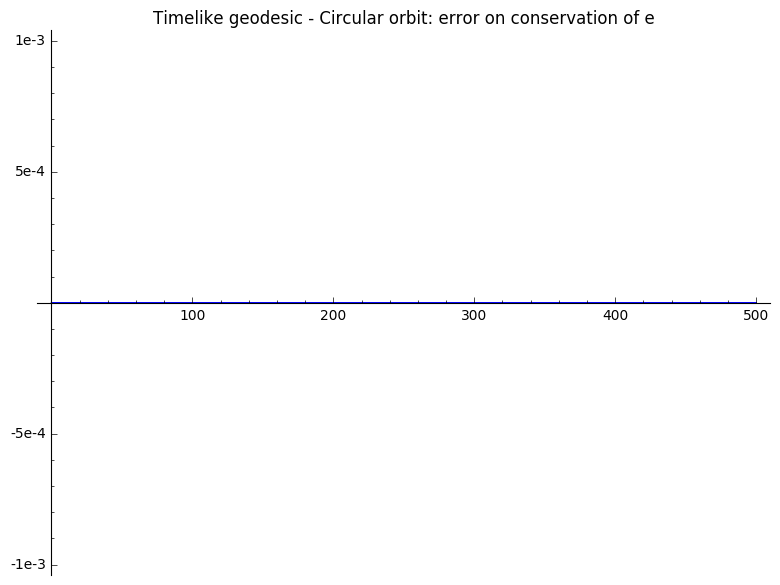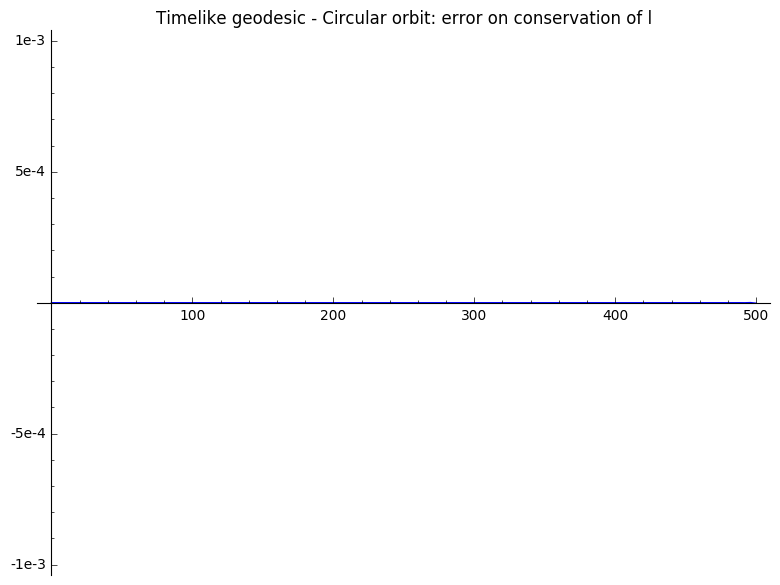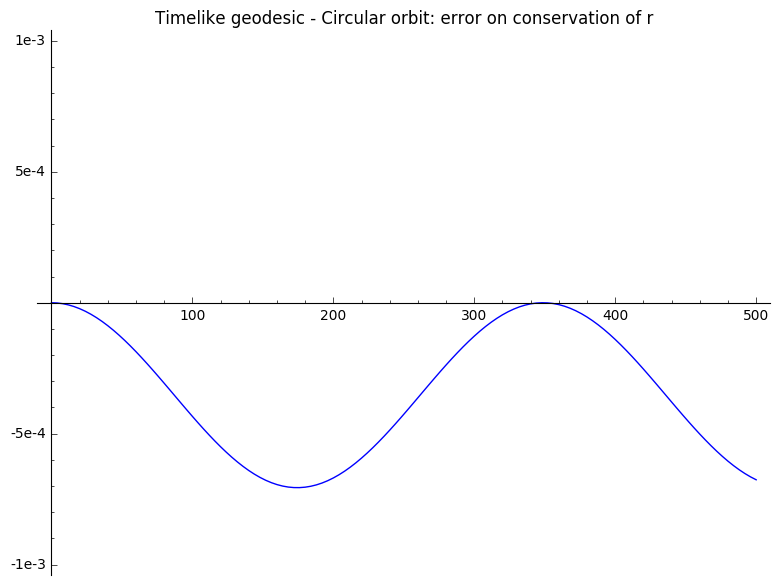The second graph, which displays the evolution of radial coordinate $r$, does confirm that the orbit is circular, up to negligible oscillations.

##### Unbounded trajectory¶

In Schwarzschild spacetime, geodesics with sufficiently high radial velocity or angular momentum may induce unbounded trajectories. An example of such a geodesic (still parametrised with proper time) which has same angular momentum as that of the geodesics considered above is provided below:

In :
params_values_unbounded = {m:1, s_0:0, s_max:100, t_0:0, r_0:4, th_0:pi/2, ph_0:0,
Dt_0:sqrt(16.405)/2, Dr_0:0.210, Dth_0:0, Dph_0:4.1/16}

sol_unbounded = geod.solve(step=1, parameters_values=params_values_unbounded,
solution_key='timelike_unbounded_equatorial', verbose=True)
interp_unbounded = geod.interpolate(solution_key='timelike_unbounded_equatorial',
interpolation_key='timelike_unbounded_equatorial',
verbose=True)

error_squar_norm_unbounded = []
error_e_unbounded = []
error_l_unbounded = []

i = 0
for (S,T,R,TH,PH) in sol_unbounded:
P = geod(S, interpolation_key='timelike_unbounded_equatorial')
V = geod.tangent_vector_eval_at(S, interpolation_key='timelike_unbounded_equatorial')

squar_norm_unbounded = numerical_approx((g.at(P)(V,V)).substitute({m:1}))
e_unbounded = numerical_approx((-g.at(P)[0,0]*V).substitute({m:1}))
l_unbounded = numerical_approx((g.at(P)[3,3]*V).substitute({m:1}))

if i == 0:
squar_norm_unbounded_0 = squar_norm_unbounded
e_unbounded_0 = e_unbounded
l_unbounded_0 = l_unbounded

error_squar_norm_unbounded += [(S,squar_norm_unbounded - squar_norm_unbounded_0)]
error_e_unbounded += [(S,e_unbounded - e_unbounded_0)]
error_l_unbounded += [(S,l_unbounded - l_unbounded_0)]

if i == 10:
squar_norm_unbounded_0 = squar_norm_unbounded
e_unbounded_0 = e_unbounded
l_unbounded_0 = l_unbounded

error_squar_norm_unbounded += [(S,squar_norm_unbounded - squar_norm_unbounded_0)]
error_e_unbounded += [(S,e_unbounded - e_unbounded_0)]
error_l_unbounded += [(S,l_unbounded - l_unbounded_0)]

i += 1

plot_error_squar_norm_unbounded = line(error_squar_norm_unbounded)
plot_error_e_unbounded = line(error_e_unbounded)
plot_error_l_unbounded = line(error_l_unbounded)

plot_error_squar_norm_unbounded.show(title=
"Timelike geodesic - Unbounded trajectory: error on " +
"conservation of squared norm",
ymin=-1e-3, ymax=1e-3)
plot_error_e_unbounded.show(title="Timelike geodesic - Unbounded trajectory: error on conservation of e",
ymin=-1e-3, ymax=1e-3)
plot_error_l_unbounded.show(title="Timelike geodesic - Unbounded trajectory: error on conservation of l",
ymin=-1e-3, ymax=1e-3)

plot3D_projected_geod_unbounded = geod.plot_integrated(interpolation_key='timelike_unbounded_equatorial',
mapping=BL_spatial_coords,
plot_points=200, thickness=2,
display_tangent=True,
plot_points_tangent=10, scale=8,
width_tangent=1, label_axes=False)

(plot3D_projected_geod_unbounded + plot3D_event_horizon + plot3D_origin).show(viewer='threejs',
online=True)

Performing numerical integration with method 'rk4_maxima'...
Numerical integration completed.

Checking all points are in the chart domain...
All points are in the chart domain.

The resulting list of points was associated with the key 'timelike_unbounded_equatorial' (if this key already referred to a former numerical solution, such a solution was erased).
Performing cubic spline interpolation by default...
Interpolation completed and associated with the key 'timelike_unbounded_equatorial' (if this key already referred to a former interpolation, such an interpolation was erased).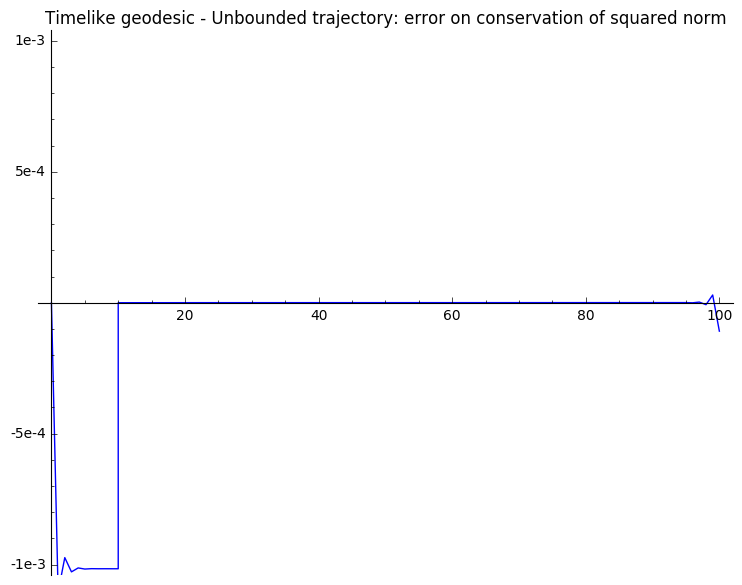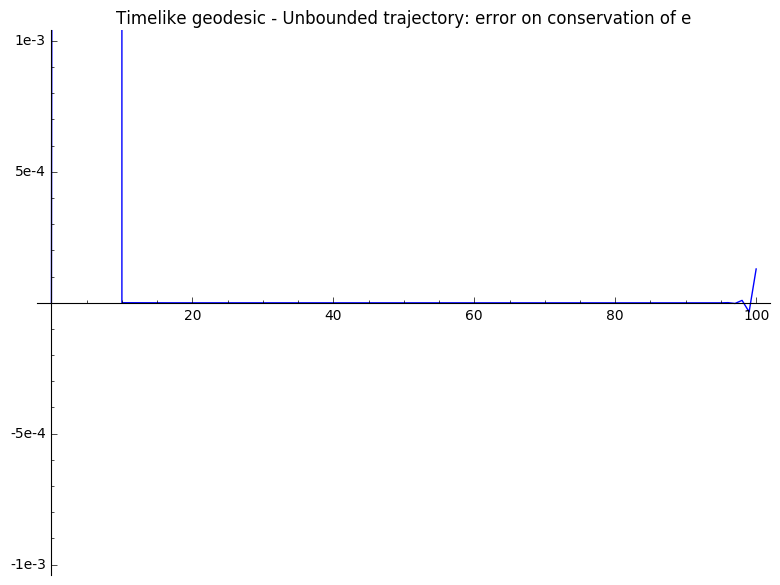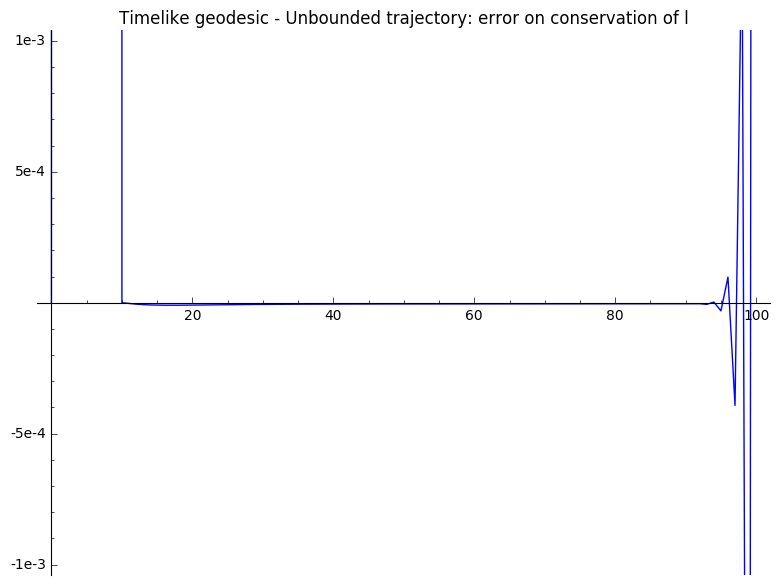#### Null geodesics¶

##### Unstable circular orbit¶

The only null, bounded geodesics of Schwarzschild spacetime that do not cross the event horizon induce unstable circular orbits. In addition, the (constant) radius of such trajectories necessarily equals $3m$.

Below is provided an example of such a (affinely parametrised) geodesic, for which conservation of squared norm, energy and angular momentum is checked:

In :
params_values_null_circular = {m:1, s_0:0, s_max:60, t_0:0, r_0:3, th_0:pi/2, ph_0:0,
Dt_0:1/sqrt(3), Dr_0:0, Dth_0:0, Dph_0:1/9}

sol_null_circular = geod.solve(step=1, parameters_values=params_values_null_circular,
solution_key='null_circular_equatorial', verbose=True)
interp_null_circular = geod.interpolate(solution_key='null_circular_equatorial',
interpolation_key='null_circular_equatorial',
verbose=True)

error_squar_norm_null_circular = []
error_e_null_circular = []
error_l_null_circular = []
error_r_null_circular = []

i = 0
for (S,T,R,TH,PH) in sol_null_circular:
P = geod(S, interpolation_key='null_circular_equatorial')
V = geod.tangent_vector_eval_at(S, interpolation_key='null_circular_equatorial')

squar_norm_null_circular = numerical_approx((g.at(P)(V,V)).substitute({m:1}))
e_null_circular = numerical_approx((-g.at(P)[0,0]*V).substitute({m:1}))
l_null_circular = numerical_approx((g.at(P)[3,3]*V).substitute({m:1}))

if i == 0:
squar_norm_null_circular_0 = squar_norm_null_circular
e_null_circular_0 = e_null_circular
l_null_circular_0 = l_null_circular
R_0 = R

error_squar_norm_null_circular += [(S, squar_norm_null_circular
- squar_norm_null_circular_0)]
error_e_null_circular += [(S, e_null_circular - e_null_circular_0)]
error_l_null_circular += [(S, l_null_circular - l_null_circular_0)]
error_r_null_circular += [(S, R - R_0)]

i += 1

plot_error_squar_norm_null_circular = line(error_squar_norm_null_circular)
plot_error_e_null_circular = line(error_e_null_circular)
plot_error_l_null_circular = line(error_l_null_circular)
plot_error_r_null_circular = line(error_r_null_circular)

plot_error_squar_norm_null_circular.show(title=
"Null geodesic - Circular orbit: error on conservation of squared norm",
ymin=-1e-3, ymax=1e-3)
plot_error_e_null_circular.show(title="Null geodesic - Circular orbit: error on conservation of e",
ymin=-1e-3, ymax=1e-3)
plot_error_l_null_circular.show(title="Null geodesic - Circular orbit: error on conservation of l",
ymin=-1e-3, ymax=1e-3)
plot_error_r_null_circular.show(title="Null geodesic - Circular orbit: error on conservation of r",
ymin=-1e-3, ymax=1e-3)

plot3D_projected_geod_null_circular = geod.plot_integrated(interpolation_key='null_circular_equatorial',
mapping=BL_spatial_coords,
plot_points=200, thickness=2,
display_tangent=True,
plot_points_tangent=10, scale=8,
width_tangent=1, label_axes=False)

(plot3D_projected_geod_null_circular + plot3D_event_horizon + plot3D_origin).show(viewer='threejs',
online=True)

Performing numerical integration with method 'rk4_maxima'...
Numerical integration completed.

Checking all points are in the chart domain...
All points are in the chart domain.

The resulting list of points was associated with the key 'null_circular_equatorial' (if this key already referred to a former numerical solution, such a solution was erased).
Performing cubic spline interpolation by default...
Interpolation completed and associated with the key 'null_circular_equatorial' (if this key already referred to a former interpolation, such an interpolation was erased).